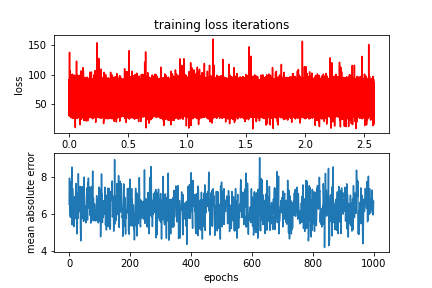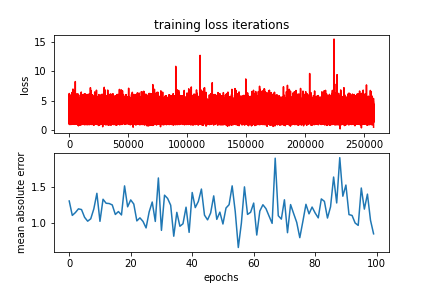# NN regression errors too high

Hi,
I’m new to pytorch. I just did a course and I wanted to try doing a DNN to predict temperatures given the parameters. I have done this using keras and I found a mse of 0.02 which is good. But when I try doing the same thing on PyTorch I get higher values of errors. I have used the code used in the course and changed it to fit the regression instead of the classification of images problem. I wanna know what I am doing wrong. Thanks.

The model class is :

``````class Net(nn.Module):

# Constructor
def __init__(self, D_in, H1, H2, D_out):
super(Net, self).__init__()
self.linear1 = nn.Linear(D_in, H1)
self.linear2 = nn.Linear(H1, H2)
self.linear3 = nn.Linear(H2, D_out)

# Prediction
def forward(self, x):
x = torch.relu(self.linear1(x))
x = torch.relu(self.linear2(x))
x = self.linear3(x)
return x

model = Net(input_size, hidden_layer_size, hidden2, output)
``````

and training the model is :

``````epochs = 100
i = 0
useful_stuff = {'training_loss': [],'validation_accuracy': []}
for epoch in range(epochs):
for i, (x, y) in enumerate(train_set):

z = model(x.view(-1,size).float())
loss = criterion(z, y.float())
loss.backward()
optimizer.step()
#loss for every iteration
useful_stuff['training_loss'].append(loss.data.item())
correct = 0
for x, y in test_set:
#validation

z = model(x.view(-1, size).float())
#print(z.detach().numpy())
abs_delta = np.abs(z.detach().numpy() - y.detach().numpy()[:, np.newaxis])

accuracy = np.mean(abs_delta)
useful_stuff['validation_accuracy'].append(accuracy)
``````

the results are here:Could you check the shapes of `z` and `y` and make sure both are equal?
If these shapes differ, internal broadcasting will be used, which will raise a warning and is sometimes ignored by the user.

Thanks!! it improved the errors but they are still higher than the keras results. why?It’s hard to tell what else might be wrong. Do you see any other warnings and could you verify that all tensor shapes are used as expected? E.g. in the `abs_delta` calculation you are using `[:, np.newaxis]` on the target while you are directly passing the target to `criterion`. Why is this the case?

when I first integrated de abs_delta I noticed that z was size [batch size, 1] and y was [1, batch size] so I did the [:, np.newaxis] to get it to the right size but I didn’t do the same with the loss part but instead of [batch size, 1 ] it was [batch size] I now fix them both with y.view(-1,1) and the error decreased.
is the error determination correct?
Shouldn’t the error decrease with epochs?
sorry for badgering you! and thanks!